## Arrays in JAVA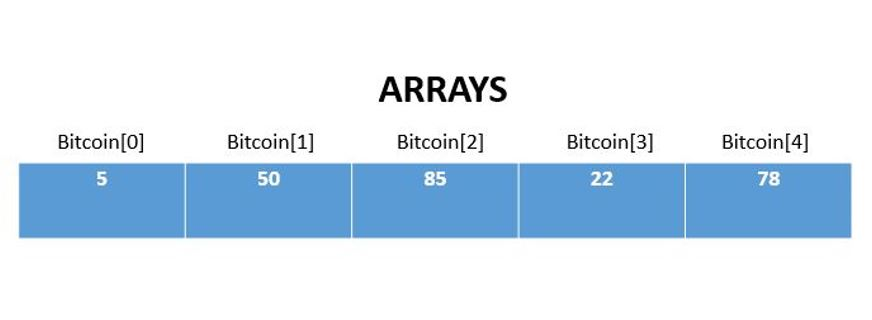An array is a collection of variables which are of similar type. Arrays can be accessed with their indexes.

Arrays can be used to store numbers, list of values and other variables.

Consider the following example below :

int[ ] Bitcoin = new int;

Here, we are defining an array Bitcoin of integer datatype consisting of 5 Bitcoins.

We can reference each element in the array with an index, say the elements of the array are {5,50,85,22,78}. We can access the 2nd element by using Bitcoin. Note indexing in Java starts at 0.

Let us look into a program to access arrays by referencing:

public class Cryptocurrency {

public static void main(String[] args) {

String[ ] Blockchain = { “Amsterdam”, “Berlin”, “Capetown”, “Delhi”};

System.out.println(Blockchain);

}

}

Program to calculate length of an array.

public class Coins {

public static void main(String[] args) {

int[ ] Bitcoin = new int;

System.out.println(Bitcoin.length);

}

}

Program to find sum of an array.

public class Coins {

public static void main(String[] args) {

int [ ] Bitcoin = {5,50,85,22,78};

int sum=0;

for(int k=0; k<Bitcoin.length; k++) {

sum += Bitcoin[k];

}

System.out.println(sum);

}

}

In this code, the variable sum stores all the variables by adding all the elements of array. Initially the sum is assigned a value of zero. A for loop traverses through the array list because of the expression k<Bitcoin.length, that is the number of elements will be equivalent to a value less than length of the array. Remember, the indexing starts at  for arrays.

Printing values of an Array.

public class Coins {

public static void main(String[] args) {

int[ ] values = {5,50,85,22,78};

for (int P: values) {

System.out.println(P);

}

}

}

2D and 3D Arrays

Accessing 2D Array

public class Coins {

public static void main(String[] args) {

int[ ][ ] Bitpart = { {77, 55, 34}, {41, 54, 63} };

int j = Bitpart;

System.out.println(j);

}

}

Accessing 3D Array

public class Coins {

public static void main(String[] args) {

int[ ][ ] Bitpart = { {17, 21, 32}, {41,70,44}, {51, 62, 75} };

Bitpart = 32;

int m = Bitpart;

System.out.println(m);

}

}

Similarly, N dimensional arrays can also be accessed.

```Source:1)THE Java™ Programming Language, Fourth Edition

By Ken Arnold, James Gosling, David Holmes

2) SoloLearn Online Platform.```

## Functions and Built-in Functions in Python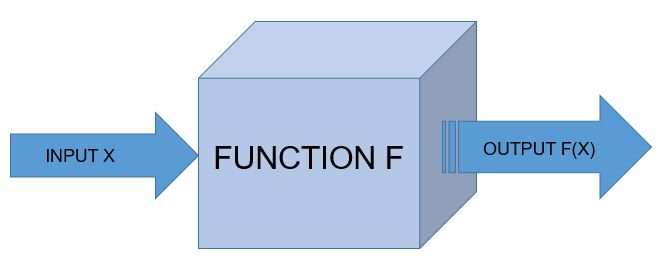Function

A function is the simplest callable object in Python.

NOTE: For Indentation either use TAB space or 4 space characters, never both.

def tax(bill):
“””Adds 25% tax to a restaurant bill.”””
bill *= 1.25
print “With tax: %f” % bill
return bill

def tip(bill):
“””Adds 35% tip to a restaurant bill.”””
bill *= 1.35
print “With tip: %f” % bill
return bill

meal_cost = 100
meal_with_tax = tax(meal_cost)
meal_with_tip = tip(meal_with_tax)

Function Syntax

“”” This is spam function”””            /* Comment*/
print “Spaceship!”                            /*body*/

spam()

Call and Response

def square(n):
“””Returns the square of a number.”””
squared = n**2
print “%d squared is %d.” % (n, squared)
return squared

square(5)

Parameters and Arguments

A function can require as many parameters as you’d like, but when called the function, it should generally pass in a matching number of arguments.

result = base**exponent
print “%d to the power of %d is %d.” % (base, exponent, result)

Functions Calling Functions

A function can call another function.

def n_plus_one(n):
return n + 1

return n_plus_one(n) + 2

Importing Module

A module is a file that contains definitions, including variables and functions, that we can use once it is imported.

import math
print math.sqrt(25)

math includes a number of useful variables and functions, and sqrt() is one of those functions. In order to access math, all you need is the import keyword. When you simply import a module this way, it’s called a generic import.

It’s possible to import only specific variables or functions from a given module. Pulling in just a single function from a module is called a function import.

# Import *just* the sqrt function from math

from math import sqrt

if we want all the variables and functions in a module but don’t want to have to constantly type math then we can use

# Import *everything* from the math module

from math import *

Built-in Functions

max()

The max() function takes any number of arguments and returns the largest one.

>> maximum = max(10,35,64)
>> print maximum

min()

min() function returns the smallest of a given series of arguments.

>> minimum = (10,35,64)
>> print minimum

abs()

The abs() function returns the absolute value of the number it takes as an argument, it gives the number’s distance from 0 on an imagined number line.
>> absolute = abs(-42)
>> print absolute

type()

The type() function returns the type of the data it receives as an argument.

>> print type(42)
>> print type(4.2)
>> print type(‘spam’)

Now let us see the code which returns biggest, smallest and absolute values.

def biggest_number(*args):
print max(args)
return max(args)

def smallest_number(*args):
print min(args)
return min(args)

def distance_from_zero(arg):
print abs(arg)
return abs(arg)

biggest_number(-105, -53, 51, 100)
smallest_number(-106, -55, 54, 105)
distance_from_zero(-104)

These are some of the functions and built-in functions in python.

## Google Analytics for Jan-Feb-March 2018(Q4 )The statistics for MOHANMA.COM for Jan-Feb-March(starting Jan 26th to 31st March) are as shown above.

There have been a total of 737 sessions with 1673 pageviews.

The Bounce Rate of the website is 66.76%, which is pretty good as lower the bounce rate better the performance of website.

New visitors account for 76.5% of total visitors and returning visitors account for 23.5%.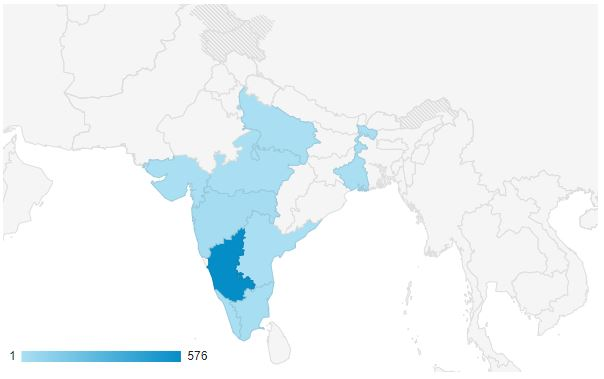India accounts for the highest traffic, with Karnataka being the leading region to in-flowing traffic with 576 sessions so far.

Among devices, mobile phones lead the traffic generated followed by desktop and lastly by tablets.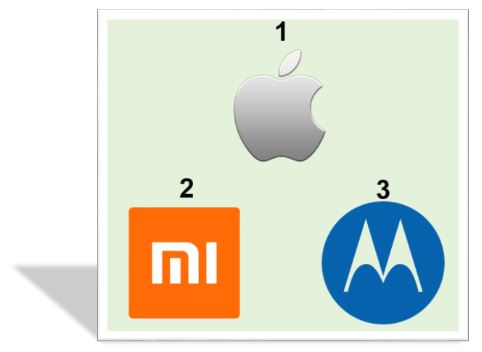Apple iphones are the leading mobile phones used to access the website followed by Xiaomi and Motorola phones.

Thanks to everyone who have been visiting the website.

Love,

Mohan M A

## Basics of Python – Part 2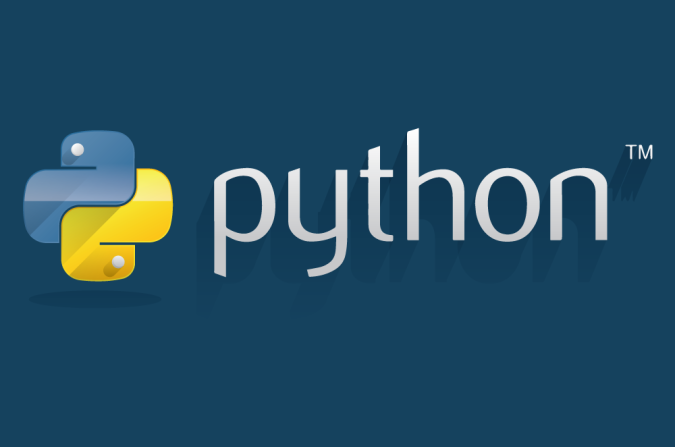Dot Notation

Using dot notation with strings.
Let us consider an example where we convert the string to upper case using dot notation.

>>> mysore = “The city is awesome !”

>>> print len(mysore)
>>> print mysore.upper()

Printing strings

>>> print(“This String”)

Printing Variables

>>> Stone_cold = “Stunner”
>>> print(Stone_cold)

Concatenation

Combining strings together is called concatenation . The + operator combine the strings.

>>> print “Indian “+ “Air ” +”Force ”

The space after the word is to make the string look like sentence.

String Conversion

The str() method converts non-strings into strings.

>>> print “Sourav Ganguly scored “+ str(100) + “on his Test Debut”

String Formatting

The % operator after a string combines a string with variables. The % operator will replace  %s in the string with the string variable.

Example 1:
>>> string_1 = “Sourav”

>>> print “Indian captain %s is also called as %s.” % (string_1, string_2)

Example 2:

>>> batsman = raw_input(“Who is the batsman?”)
>>> captain = raw_input(“Who is the captain?”)
>>> series = raw_input(“Which series did he win for India?”)

>>> print “The Indian %s and %s Sourav Ganguly won %s series for India.” % (batsman, captain, series)

Date and Time

We can import datetime function from datetime module.

>>> from datetime import datetime

Current Date and Time

Here is the code to print current date and time.

>>> from datetime import datetime
>>> now = datetime.now()
>>> print datetime.now()

Control Flow

The ability to choose from different situations based on what’s happening in the program.

>>> x = int(input(“Please enter an integer: “))

>>> if x < 0:

…     x = 0

…     print(‘Negative changed to zero’)

… elif x == 0:

…     print(‘Zero’)

… elif x == 1:

…     print(‘Single’)

… else:

…     print(‘More’)

if statements, for statements, range fuction, break and continue, else clause loops, pass statements are few of the control flow statements.

Comparators

Equal to (==)
Not equal to (!=)
Less than (<)
Less than or equal to (<=)
Greater than (>)
Greater than or equal to (>=)

Boolean Operators

AND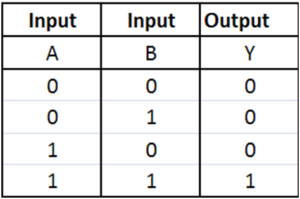OR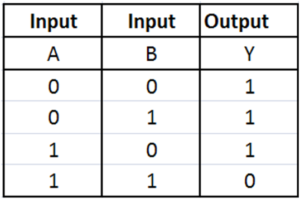NOT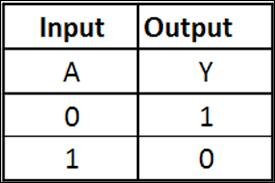Conditional Syntax

The statements used for decision making are called conditional statements.

if condition_1:

statement_block_1

elif condition_2:

statement_block_2

else:

statement_block_3

This post concludes basics of python.